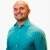# SAT Prep How to determine the length of a parallelogram given a side and perimeterThis lesson will look at an example problem involving perimeter. This lesson will give an explanation of how to determine length of a parallelogram given a side and perimeter with a step by step look to... This lesson will look at an example problem involving perimeter. This lesson will give an explanation of how to determine length of a parallelogram given a side and perimeter with a step by step look to better explain the concept.
More... Collapse
11 Views